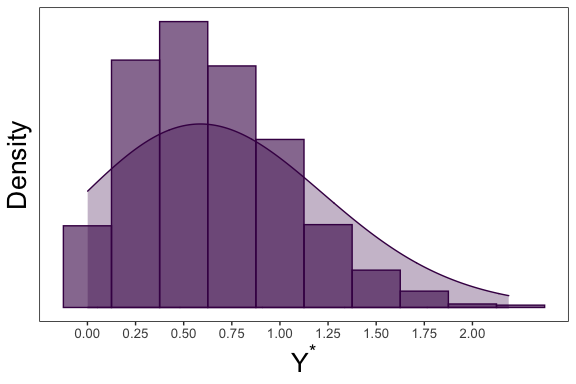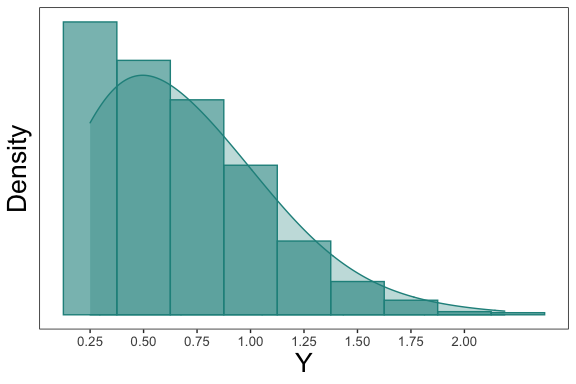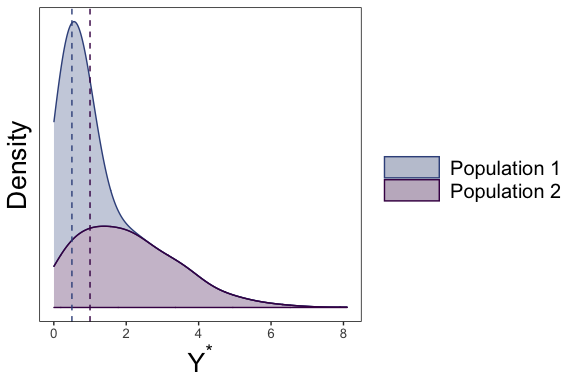# tcensReg: An Introduction

#### 2020-06-29

The goal of this package is to estimate parameters from a linear model when the data comes from a truncated normal distribution with censoring. Maximum likelihood values are returned. There are multiple method available for optimization with the default set as conjugate gradient. This package is also able to return maximum likelihood estimates for truncated only or censored only data similar to truncreg and censReg packages.

# Installation

You can install tcensReg from CRAN for the stable release or install the GitHub version for the active development version:

#stable CRAN version
install.packages("tcensReg")

# or ----

#active devel. GitHub version
install.packages("devtools")
devtools::install_github("williazo/tcensReg")

# Example 1: Single Population

Some common examples where this type of problem may arise is when there is a natural truncation imposed by the structure of the data. For instance several applications have an implied zero truncation such as product lifetimes, age, or detection thresholds. To show how to implement the functions within the package, I will demonstrate a simple simulation example.

Assume that we have observations from an underlying truncated normal distribution. In our case we will assume a zero-truncated model by setting a=0. We generate this truncated normal data below and refer to it as y_star.

#loading the package
library(tcensReg)
mu <- 0.5
sigma <- 0.5
a <- 0
#generate random values from the truncated normal distribution using tcensReg function
y_star <- rtnorm(n=1000, mu=mu, sd=sigma, a=a)
#note that the lowerbound will always be non-negative
round(range(y_star), 3)
##  0.001 2.189

Next, we can imagine a scenario where we have an imprecise measurement of y_star leading to censoring. In our case we assume that values below a limit of detection, nu, are censored. This creates a random variable y.

In the example below we set our limit of detection as nu=0.25.

nu <- 0.25
y <- ifelse(y_star <= nu, nu, y_star)
#calculating the number of censored observations
sum(y == nu)/length(y) 
##  0.174
#collecting the uncensored and censored data together
dt <- data.frame(y_star, y) 

We can observe the histogram and density plot for the uncensored data, which shows the zero-truncation.We can then compare this to the censored observations belowWe can then estimate the mean, mu, and standard deviation, sigma, using y with the tcensReg package as shown below.

#loading the package
library(tcensReg)
t_mod <- tcensReg(y ~ 1, data=dt, a=0, v=0.25)
summary(t_mod)
##
## Call:
## tcensReg(formula = y ~ 1, a = 0, v = 0.25, data = dt)
##
## Assumed Distribution:
## Truncated Normal with Censoring
##
## Count of Observations:
##    Total Observations Censored Observations
##                  1000                   174
##
##
## Coefficients:
##             Estimate Std. Error t value
## (Intercept)   0.4902     0.0305 16.0765
## sigma         0.5092     0.0139 36.6421
##
## Log Likelihood: -657.218
## Information Criterion: AIC=1318.4359 BIC=1328.2514
## Optimization Method: CG
## Psuedo R2: 0 method - mckelvey_zavoina

By default the coefficients are returned along with log likelihood and other fit criterion statistics. Note that the Pseudo R2 in the case of an intercept only model is exactly equal to zero.

names(t_mod)
##   "theta"             "convergence"       "initial_ll"
##   "final_ll"          "var_cov"           "method"
##   "info_criteria"     "model_matrix"      "call"
##  "n_count"           "latent_assumption"

Note that the this object contains parameter estimates theta, convergence criterion code, initial/final log-likelihood values, variance-covariance matrix, method of optimization, information criterion, design matrix used from the model, formula call, count of total/censored observations, and latent distributional assumption.

Comparing the values to the truth we see that the estimates are unbiased.

#tcensReg model
output <- tcensReg(y ~ 1, data=dt, a=a, v=nu)
#extracting the point estimates
tcensReg_est <- coef(output) #this returns sigma rather than log sigma

#OLS model
lm_output <- lm(y ~ 1, data=dt)
lm_est <- c(coef(lm_output), summary(lm_output)$sigma) #censored only model, i.e., Tobit model cens_output <- tcensReg(y ~ 1, data=dt, v=nu)  ## Warning: a is not specified indicating no truncation cens_est <- coef(cens_output) results_df <- data.frame(rbind(c(mu, sigma), t(tcensReg_est), lm_est, t(cens_est))) names(results_df) <- c("mu", "sigma") row.names(results_df) <- c("Truth", "tcensReg", "Normal MLE", "Tobit") results_df$mu_bias <- results_df$mu - mu results_df$sigma_bias <- results_df$sigma - sigma knitr::kable(results_df, format="markdown", digits=4) mu sigma mu_bias sigma_bias Truth 0.5000 0.5000 0.0000 0.0000 tcensReg 0.4902 0.5092 -0.0098 0.0092 Normal MLE 0.6643 0.3751 0.1643 -0.1249 Tobit 0.6219 0.4375 0.1219 -0.0625 Other methods result in significant bias for both mu and sigma. # Example 2: Two Population Model with Separate Variances As an extension for the single population model above, we can imagine a two independent truncated normal random variables that have common censoring and truncation values but different standard deviations. We can simulate the underlying truncated normal distributions Y1_star and Y2_star similar to $$Y$$ above except now we allow them to have separate mean and variances. For this example we let mu_1=0.5, mu_2=1, sigma_1=0.25, sigma_2=2, and a=0. mu_1 <- 0.5 mu_2 <- 1 sigma_1 <- 0.25 sigma_2 <- 2 a <- 0 set.seed(032420) y_1_star <- rtnorm(1000, mu = mu_1, sd = sigma_1, a = a) y_2_star <- rtnorm(1000, mu = mu_2, sd = sigma_2, a = a) df <- data.frame(y_star = c(y_1_star, y_2_star), group = c(rep("Population 1", length(y_1_star)), rep("Population 2", length(y_2_star)))) Plotting each of these uncensored population densities, we can see the difference in shape based on the underlying parameter selection.Then censoring each observation at nu, we are left with Y1 and Y2. Again, we let nu=0.25. nu <- 0.25 df$y <- ifelse(df$y_star<=nu, nu, df$y_star)

We then can fit our model with separate variances for each group using the command tcensReg_sepvar as shown below.

mod_result <- tcensReg_sepvar(y ~ group, a=a, v=nu, group_var="group", method="maxLik", data=df)
mod_result
## $theta ## (Intercept) groupPopulation 2 log_sigma1 log_sigma2 ## 0.4933551 0.6659116 -1.3281990 0.6546893 ## ##$convergence
##  2
##
## $initial_ll ##  -2013.046 ## ##$final_ll
##  -1933.59
##
## $var_cov ## (Intercept) groupPopulation 2 log_sigma1 log_sigma2 ## (Intercept) 9.384525e-05 -9.384525e-05 -9.865163e-05 -1.179984e-14 ## groupPopulation 2 -9.384525e-05 2.475291e-02 9.865163e-05 -6.214006e-03 ## log_sigma1 -9.865163e-05 9.865163e-05 8.721167e-04 1.240418e-14 ## log_sigma2 -1.179984e-14 -6.214006e-03 1.240418e-14 2.211993e-03 ## ##$method
##  "maxLik"
sepvar_est <- mod_result$theta mu_1_est <- sepvar_est mu_2_est <- sum(sepvar_est[1:2]) sigma_1_est <- exp(sepvar_est) sigma_2_est <- exp(sepvar_est) results_df <- data.frame(rbind(c(mu_1, mu_2, sigma_1, sigma_2), c(mu_1_est, mu_2_est, sigma_1_est, sigma_2_est))) names(results_df) <- c("mu_1", "mu_2", "sigma_1", "sigma_2") row.names(results_df) <- c("Truth", "tcensReg") results_df$mu1_pct_bias <- paste0(round(((results_df$mu_1 - mu_1)/mu_1)*100, 2), "%") results_df$mu2_pct_bias <- paste0(round(((results_df$mu_2 - mu_2)/mu_2)*100, 2), "%") results_df$sigma1_pct_bias <- paste0(round(((results_df$sigma_1 - sigma_1)/sigma_1)*100, 2), "%") results_df$sigma2_pct_bias <- paste0(round(((results_df$sigma_2 - sigma_2)/sigma_2)*100, 2), "%") knitr::kable(results_df, format="markdown", digits=4) mu_1 mu_2 sigma_1 sigma_2 mu1_pct_bias mu2_pct_bias sigma1_pct_bias sigma2_pct_bias Truth 0.5000 1.0000 0.250 2.0000 0% 0% 0% 0% tcensReg 0.4934 1.1593 0.265 1.9245 -1.33% 15.93% 5.98% -3.77% # Performance Comparison: Censored-Only and Truncated-Only (Intercept Only Model) Note also that the tcensReg can also estimate parameters in the censored-only or truncated-only cases. We show below that by using analytic values in the tcensReg implementation that our method is faster then the alternative estimation procedures while providing better variance estimates. With a small set of covariates and p<<n we can use the Newton Raphson method of optimization, which is computationally fast with few covariates. library(microbenchmark) #testing the censored-only regression library(censReg) cens <- microbenchmark(tcensReg_method = tcensReg(y ~ 1, data=dt, v=nu, method="Newton"), censReg_method = censReg(y ~ 1, left=nu, data=dt)) knitr::kable(summary(cens), format="markdown", digits=4) expr min lq mean median uq max neval cld tcensReg_method 5.3200 5.4625 6.7331 5.6227 6.2430 18.8487 100 a censReg_method 14.4658 15.0461 18.6427 16.2989 22.1891 38.3765 100 b #point estimates are equivalent tcensReg_est <- as.numeric(tcensReg(y ~ 1, data=dt, v=nu, method="Newton")$theta)
censReg_est <- as.numeric(coef(censReg(y ~ 1, left=nu, data=dt)))
all.equal(tcensReg_est, censReg_est)
##  TRUE
#testing the truncated-only regression
library(truncreg)
trunc <- microbenchmark(
tcensReg_method = tcensReg(y_star ~ 1, data=dt, a=a, method="Newton"),
truncreg_method = truncreg(y_star ~ 1, point=a, data=dt))
knitr::kable(summary(trunc), format="markdown", digits=4)
expr min lq mean median uq max neval cld
tcensReg_method 9.2338 9.7151 11.7873 10.1253 12.7724 29.4476 100 a
truncreg_method 28.0528 29.0570 34.5233 33.4165 36.4283 82.5259 100 b
tcensReg_est <- as.numeric(tcensReg(y_star ~ 1, data=dt, a=a, method="Newton")\$theta)
#note truncreg returns sigma not log_sigma so we need to exponentiate our value
tcensReg_est <- exp(tcensReg_est)
truncreg_est <- as.numeric(coef(truncreg(y_star ~ 1, point=a, data=dt)))
all.equal(tcensReg_est, truncreg_est)
##  "Mean relative difference: 8.402706e-08"